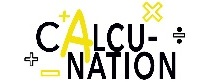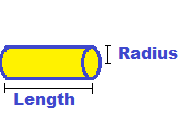Math isn't easy for everyone,
CalcuNation is.

# Cylinder Surface Area Calculator

## Find the surface area of a cylinder with this Cylinder Surface Area Calculator.Length:
Fill in the radius of the base and the length of the cylinder to determine
the total surface area. (including the circle area on both ends)

### How do you find the surface area of a cylinder?

To find the surface area, use this cylinder surface area formula:
Cylinder Surface Area = 2 x (3.1416 x Radius2) +
(2 x 3.1416 x Radius x Length)Example: The surface area of a cylinder with base radius of 3 inches
and length of 5 inches:
Cylinder Surface Area = 2 x (3.1416 x 32) + (2 x 3.1416 x 3 x 5)

Calculated out this gives a total surface area of 150.7968 Square Inches.